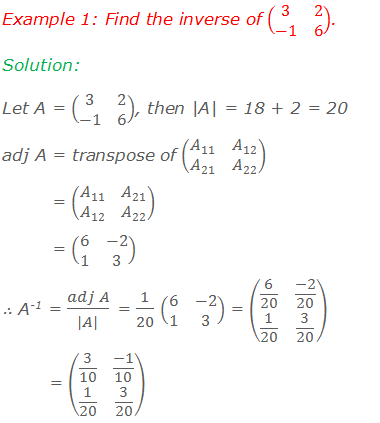Let A be a square matrix, and Aij be the cofactors of the elements aij of the matrix A, then adjoint or adjugate of A denoted by adj A is the matrix obtained by transposing the matrix of cofactors of A.#### For example:### Inverse of Matrices by Adjoint Method### Worked Out Examples#### Do you have any questions regarding the adjoint of a matrix?

You can ask your questions or problems here, in the comment section below.